Understanding Fixed-Income Risk and Return | IFT World
IFT Notes for Level I CFA® Program
IFT Notes for Level I CFA® Program

# Part 3

## 6. Properties of Bond Duration

The input variables for determining Macaulay and modified yield duration of fixed-rate bonds are:

• Coupon rate or payment per period
• Yield-to-maturity per period
• Number of periods to maturity
• Fraction of the period that has gone by

By changing one of the above variables while holding others constant, we can analyze the properties of bond duration, which in turn, helps us assess the interest rate risk. We will use the formula for Macaulay duration to understand the relationship between each variable and duration: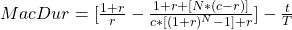The fraction of the coupon period that has gone by (t/T)

First, let us consider the relationship between fraction of time that has gone by (t/T) and duration. There is no change to the expression in braces {} as time passes by. Fraction of time (t/T) increases as time passes by. Assume T is 180. If 50 days have passed, then t/T = 0.277. If 90 days have passed, then t/T = 0.5. If 150 days have passed, then t/T = 0.83. As t/T increases from t = 0 to t = T with passing time, MacDuration decreases in value. Once the coupon is paid, t/T becomes zero and MacDuration jumps in value. When time to maturity is plotted against MacDuration, it creates a saw tooth pattern as shown graphically below:

Source: CFA Program Curriculum, Understanding Fixed-Income Risk and Return

Interpretation of the Macaulay duration between coupon payments with a constant yield to maturity:

• Note: Read the diagram from right to left. As time passes between coupon periods, duration decreases in value.
• Once the coupon is paid, it jumps back up creating a saw tooth pattern.

Now, we look at the relationship between Macaulay duration and change in the coupon rate, yield to maturity, and the time to maturity. This is depicted in the exhibit below:

Properties of the Macaulay Yield Duration

Time-to-Maturity

The relationship between Macaulay Duration and time-to-maturity for four types of bonds (zero-coupon, discount, premium, and perpetuity) at t/T = 0 are shown.

• Zero-coupon bond: Pays no coupon so c = 0. Plugging in c = 0 and t/T = 0 in MacDur formula, we get Macaulay Duration = N. For a zero-coupon bond, duration is its time-to-maturity.
• Perpetuity: A perpetual bond is one that does not mature. There is no principal to redeem. It makes a fixed coupon payment forever. If N is a large number in the above equation, then the second part of the expression in the braces becomes zero. Denominator: N is an exponent.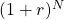is a very large number. So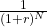must be zero and the value in the numerator will not matter here. Macaulay duration of a perpetual bond is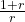as N approaches infinity.
• Premium bond: Bonds are trading at a premium above par or at par. The coupon rate is greater than or equal to yield-to-maturity (r). The numerator of the second expression in braces is always positive because c-r is positive. The denominator of the second expression in braces is always positive. Second expression as a whole is always positive. MacDuration = less thanbecause the second expression in braces is positive. As time passes, it approaches.
• Discount bond: For a discount bond, the coupon rate is below yield-to-maturity. The Macaulay Duration increases for a longer time-to-maturity. The numerator of the second expression in braces is negative because c-r is negative. Put together, duration at some point exceeds, reaches a maximum and approaches(the threshold) from above. This happens when N is large and coupon rate (c) is below the yield-to-maturity (r). As a result, for a long-term discount bond, interest rate risk can be lesser than a shorter-term bond.

The above points are summarized below:

 Relationship between bond duration and other input parameters Bond parameter Effect on Duration Higher coupon rate Lower Higher yield-to-maturity Lower Longer time-to-maturity Higher for a premium bond. Usually, holds true for a discount bond, but there can be exceptions. Exception: low coupon (relative to YTM) bond with long maturity.

Example 11: Calculating the approximate modified duration

A mutual fund specializes in investments in sovereign debt. The mutual fund plans to take a position on one of these available bonds.

 Bond Time-to-Maturity Coupon Rate Price Yield-to-Maturity (A) 5 years 10% 70.093879 20% (B) 10 years 10% 58.075279 20% (C) 15 years 10% 53.245274 20%

The coupon payments are annual. The yields-to-maturity are effective annual rates. The prices are per 100 of par value.

1. Compute the approximate modified duration of each of the three bonds using a 5 bps change in the yield-to-maturity and keeping precision to six decimals (because approximate duration statistics are very sensitive to rounding).
2. Which of the three bonds is expected to have the highest percentage price increase if the yield-to-maturity on each decreases by the same amount – for instance, by 10 bps from 20% to 19.90%?

Solution to 1:

Calculate PV+ and PV; then calculate modified duration.

Bond A:

PV= 70.093879;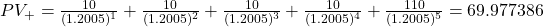PV+ = 69.977386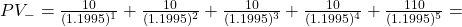70.210641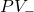= 70.210641

ApproxModDur =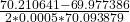= 3.33.

The approximate modified duration of Bond A is 3.33.

Bond B:

PV= 58.075279

PV+ =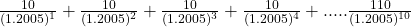= 57.937075

PV+ = 57.937075

PV=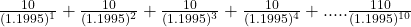= 58.213993

PV= 58.213993

ApproxModDur =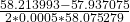= 4.77

The approximate modified duration of Bond B is 4.77.

Bond C:

PV= 53.245274

PV+ =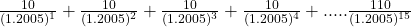= 53.108412

PV+ = 53.108412

PV =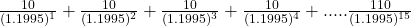= 53.382753

PV = 53.382753

ApproxModDur =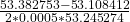= 5.15.

The approximate modified duration of Bond C is 5.15.

Solution to 2:

Bond C with 15 years-to-maturity has the highest modified duration. If the yield to maturity on each is decreased by the same amount – for instance, by 10bps, from 20% to 19.90% – Bond C would be expected to have the highest percentage price increase because it has the highest modified duration.

Interest Rate Risk Characteristics of a Callable Bond

A callable bond is one that might be called by the issuer before maturity. This makes the cash flows uncertain, so the YTM cannot be determined with certainty. The exhibit below plots the price-yield curve for a non-callable/straight bond and a callable bond. It also plots the change in price for a change in the benchmark yield curve. Bond price is plotted on the y-axis and the benchmark yield on the horizontal axis.

Interpretation of the diagram:

• The two bonds share the same features: credit risk, coupon rate, payment frequency, and time to maturity, but their prices are different when interest rates are low.
• When interest rates are low (left side of the graph), the price of the non-callable bond is always greater than the callable bond.
• The difference between the non-callable bond and callable bond is the value of the embedded call option.
• The call option is more valuable at low interest rates because the issuer has an option to refinance debt at lower interest rates than paying a higher interest to existing investors. For instance, if a bond is callable at 102% of par, then its price cannot increase beyond 102 of par even if interest rates decrease. This is the reason behind the callable bond’s negative convexity.
• From an investor’s perspective a call option is risky. At low interest rates if the issuer calls the bond (meaning the issuer pays back the money borrowed), then investors must reinvest the proceeds at lower rates. Notice that at higher interest rates, there is not much of a difference in price because the probability of calling the bond is less.
• At relatively low interest rates, the effective duration of a callable bond is lower than that of non-callable bond, i.e., interest rate sensitivity is low.

Interest Rate Risk Characteristics of Putable Bond

Interest Rate Risk Characteristics of Putable Bond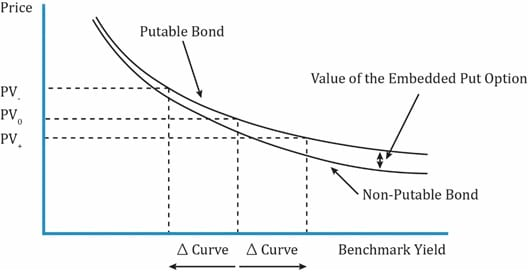Source: CFA Program Curriculum, Understanding Fixed-Income Risk and Return

Interpretation of the diagram:

• The diagram above shows the price-yield curve for a putable bond and a straight bond.
• A putable bond allows the investor to sell the bond back to the issuer; usually at par.
• At low interest rates, there is not much of a difference between a straight bond and putable bond.
• At high interest rates, the difference between the putable bond and straight bond is the value of the put option.
• When interest rates rise, the price of the bond decreases. Investors buy putable bonds to protect against falling prices as rates rise.
• The value of the put option increases as rates rise. This also limits the sensitivity of the bond price to changes in benchmark rates.

## 7. Duration of a Bond Portfolio

In the previous section, we saw how to calculate the duration for an individual bond. What if a portfolio consists of a number of bonds, how will its duration be calculated?

There are two ways to calculate the duration of a bond portfolio:

• Weighted average of time to receipt of the aggregate cash flows.
• Weighted average of the individual bond durations that comprise the portfolio.

The key points related to each method are outlined in the table below.

 Weighted Average Time to Receipt of Aggregate Cash Flows Weighted Average of Individual Bond Durations in Portfolio Theoretically correct, but difficult to use in practice. Commonly used in practice. Cash flow yield not commonly used. Cash flow yield is the IRR on a series of cash flows. Easy to use as a measure of interest rate risk. Amount and timing of cash flows might not be known because some of these bonds may be MBS, or with call options. More accurate as difference in YTMs of bonds in portfolio become smaller. Interest rate risk is usually expressed as a change in benchmark interest rates, not as a change in the cash flow yield. Assumes parallel shifts in the yield curve, i.e., all rates change by the same amount in the same direction. That seldom happens in reality. Change in the cash flow yield is not necessarily the same amount as the change in yields to maturity on the individual bonds.

Example 12: Calculating portfolio duration

An investment fund owns the following portfolio of three fixed-rate government bonds:

 Bond A Bond B Bond C Par value 15,000,000 20,000,000 40,000,000 Market value 14,769,542 25,650,379 43,796,854 Modified duration 4.328 5.643 7.210

The total market value of the portfolio is 84,216,775. Each bond is on a coupon date so that there is no accrued interest. The market values are the full prices given the par value.

1. Calculate the average modified duration for the portfolio using the shares of market value as the weights.
2. Estimate the percentage loss in the portfolio’s market value if the yield to maturity on each bond goes up by 10 bps.

Solution to 1:

The average modified duration of the portfolio is 6.23.

(14,769,542/84,216,775) × 4.328 + (25,650,379/84,216,775) × 5.643 + (43,796,854/84,216,775) × 7.210 = 6.23.

Solution to 2:

The estimated decline in market value if each yield rises by 10 bps is 0.623%. -6.23 × 0.001 = -0.00623.